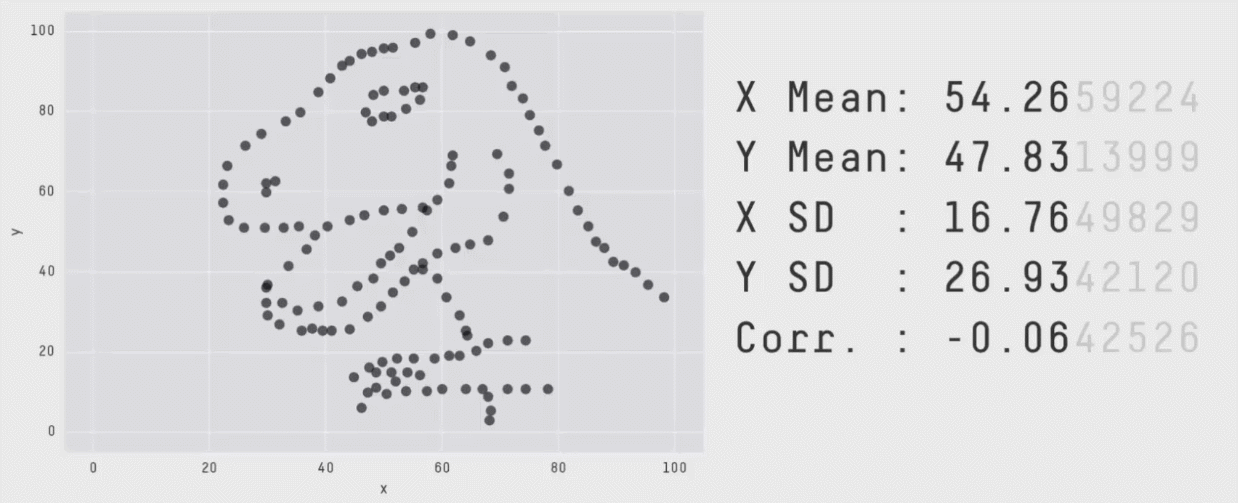# Same Stats! Different Graphs! The importance of Data Visualization.In a typical Lean Six Sigma DMAIC project, the process starts by framing the problem with regard to the process inputs and outputs that need to be measured; the data is then collected and managed using for example; MSA (Measurement System Analysis) and other data management methods. Once the data is clean and free of large measurement errors, visualization methods are used to uncover root causes.The following is an excellent reminder of why it is so important to understand your data and visual review the output as well, before drawing any conclusions.

Researchers at AutoDesk looked at multiple data sets while different in appearance, each has the same summary statistics (mean, std. deviation, and Pearson’s corner.) to 2 decimal places.

Example 1: “Datasaurus” is the initial dataset and produces “normal” summary statistics, the resulting plot is a picture of a dinosaur and create other datasets with the same summary statistics.

x͞ =54.26, y͞ = 47.83, SDx = 16.76, SDy = 26.93, Pearson’s r = -0.06Example 2: A common tool, the “Tukey Boxplot,” presents the 1st quartile, median, and 3rd quartile values on the “box,” with the “whiskers” showing the location of the furthest data points within 1.5 interquartile ranges (IQR) from the 1st and 3rd quartiles. Starting with the data in a normal distribution, and then perturbed, while ensuring that the boxplot statistics remain constant produces the results are shown below.Based on original work by Justin Matejka and George Fitzmaurice

Tags: